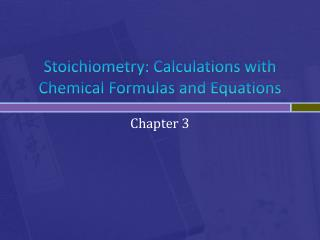DownloadDownload PresentationStoichiometry : Calculations with Chemical Formulas and Equations

# Stoichiometry : Calculations with Chemical Formulas and Equations

Télécharger la présentation## Stoichiometry : Calculations with Chemical Formulas and Equations

- - - - - - - - - - - - - - - - - - - - - - - - - - - E N D - - - - - - - - - - - - - - - - - - - - - - - - - - -
##### Presentation Transcript

1. Stoichiometry: Calculations with Chemical Formulas and Equations Chapter 3

2. 3.1 Chemical Equations • Reactants are left of the arrow • Products are right of the arrow • The symbol D is placed above the arrow to indicate that the rxn is being heated • Must have an equal number of atoms of each element on each side of the arrow • Remember you can change coefficients but NEVER change the subscripts • State of substance sometimes shown • Solid (s), liquid (l), gas (g), aqueous (aq)

3. 3.2 Patterns of Chemical Reactivity • Combination (synthesis) reactions • 2+ substances react to form one product • 2 Mg(s) + O2(g)  2 MgO(s) • Decomposition reactions • One substance reacts to product 2+ substances • CaCO3(s)  CaO(s) + CO2(g) • Combustion • Reactions that produce a flame • Most have O2 as reactant • Combustion of hydrocarbons and oxygen containing hydrocarbon derivatives generates CO2 and H2O • If insufficient O2 present will form CO in addition to CO2

4. 3.3 Formula Weights • Formula weight- sum of the atomic weights of each atom in the chemical formula • Aka molecular weight of molecules • Percent Composition- calculate the % of each element in the total mass of the compound (# of atoms of element)(atomic mass of element) x 100 Total mass of the compound

5. 3.4 Avogadro’s Number and the Mole • 6.02 x 1023 units • An element’s atomic mass expressed in grams contains 1 mole of atoms- molar mass • Going to moles divide, away from moles multiply Mass Moles Number of particles Molar mass 6.02 x 1023 22.4 L Volume (gas)

6. 3.5 Empirical Formulas from Analyses • Determining empirical from % composition • Convert % to mass (assume 100g) • Calculate moles of each element • Determine mole ratios (divide all moles by smallest #) • Multiply if needed to ensure all whole #’s • Molecular Formula from empirical Whole- number multiple = molecular weight Empirical formula weight • Multiply empirical by multiple to get molecular formula

7. Combustion analysis • Calculate the mass of C and H • Moles of C per mole CO2 • Moles of H per mole H2O • Determine mass of O • Mass of O = mass of sample – (mass of C + mass of H) • Calculate moles of C, H, O • Divide by lowest to determine ratio

8. 3.6 Stoichiometry

9. 3.7 Limiting Reactants • Substance that controls the amount of product that can form • Solving: • Convert grams to moles • Divide by coefficient in balanced equation • Smallest # is limiting reactant • Stoichiometry problem- use limiting reactant

10. Theoretical yield • Quantity of product calculated to form when all of the limiting reactant reacts • Actual yield • Amount of product actually obtained • Less than theoretical • Percent yield Actual yield x 100 Theoretical yield • Goal = close to 100%